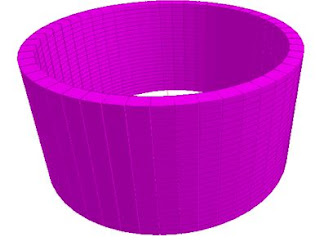## Sunday, April 21, 2019

Water tanks are usually rectangular or cylindrical in shape. Cylindrical tanks are usually employed for surface or elevated water tanks. Some of the challenges involved with cylindrical tanks are the difficulty associated with construction such as setting out, formwork preparation/installation, reinforcement installation, increased labour cost, etc.

Cylindrical tanks are subjected to radial pressure from the stored water, and/or from the retained earth when they are buried under the ground. Just like rectangular water tanks, the analysis of cylindrical tanks is easily done with the use of coefficients picked from already made tables (see Fig 2.0). The values of the coefficients are usually based on the support condition of the wall relative to the base.

The internal forces normally analysed for are;

• Circumferential tension (hoop tension)
• Vertical moments

If the wall is supported on the base in such a way that no radial movement can occur, radial shear and vertical bending result, and the circumferential tension is always zero at the bottom of the wall. This is usually referred to as the fixed joint condition, and has been considered in this publication.

Table 1.0: Coefficients for Analysis of Cylindrical Tanks Fixed at the Base (Reynolds and Steedman, 2008)
Analysis Example
Determine the maximum service values for circumferential (hoop) tension, vertical moment and radial shear in the wall of a cylindrical tank that is free at the top edge and fixed at the bottom. The wall is 400 mm thick, the tank is 4 m deep, the diameter is 8 m, and the water level is taken to the top of the wall.

Hydrostatic pressure at the base of the tank (n) = 10 kN/m3 × 4 m = 40 kN/m2
From Table 1.0;
lz2/Dh = 42/(8 × 0.4) = 5
Maximum hoop tension (t) = αtnr = 0.477 × 40 × 4 = 76.32 kN/mMaximum vertical negative moment (outside face) = αmnlz2 = 0.0059 × 40 × 42 = 3.776 kNm/m
>Maximum vertical positive moment (inside face) = αmnlz2 = 0.0222 × 40 × 42 = 14.208 kNm/m
Radial shear V = αvnlz = 0.213 × 40 × 4 = 34.08 kN/m

When analysed on Staad Pro as a concrete cylindrical tank with poisson ratio of 0.2, fixed at the bottom, and subjected to hydrostatic pressure of 40 kN/m2 the following results were obtained;Fig 2.0: Finite Element Meshing of Cylindrical TankFig 3.0: Horizontal Moment on the Tank WallsFig 4.0: Vertical Bending Moment on the Tank Walls
Table 2.0: Analysis Result from Staad Pro

Maximum vertical bending moment = 10.714 kNm/m
Maximum vertical shear stress = 0.073 N/mm2 = 29.2 kN/m
Maximum hoop stress (membrane) = 0.195 N/mm2 = 78 kN/m
Let us compare the results from Staad Pro with results from the use of coefficients (see Table 3.0).

Table 3.0: Comprison of Analysis Result from Staad Pro and use of coefficients

Thank you for visiting Structville today, and God bless you.

1.2.